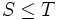# Translate-containment quasiorder

The translate-containment quasiorder is a quasiorder defined on the elements of a group as follows: for subsets$S$ and$T$ of a group$G$,$S \le T$ if and only if there exist elements$x,y \in G$ such that$xSy \subseteq T$.